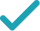Type a math problemSolve for x (complex solution)Steps Using the Quadratic Formula
Steps for Completing the Square
All equations of the form can be solved using the quadratic formula: . The quadratic formula gives two solutions, one when is addition and one when it is subtraction.
Subtract from both sides of the equation.
Subtracting from itself leaves .
This equation is in standard form: . Substitute for , for , and for in the quadratic formula, .
Square .
Multiply times .
Multiply times .
Take the square root of .
Multiply times .
Now solve the equation when is plus. Add to .
Divide by .
Now solve the equation when is minus. Subtract from .
Divide by .
The equation is now solved.
GraphGiving is as easy as 1, 2, 3
Get 1,000 points to donate to a school of your choice when you join Give With Bing
-2x^{2}+x=8
All equations of the form ax^{2}+bx+c=0 can be solved using the quadratic formula: \frac{-b±\sqrt{b^{2}-4ac}}{2a}. The quadratic formula gives two solutions, one when ± is addition and one when it is subtraction.
-2x^{2}+x-8=8-8
Subtract 8 from both sides of the equation.
-2x^{2}+x-8=0
Subtracting 8 from itself leaves 0.
x=\frac{-1±\sqrt{1^{2}-4\left(-2\right)\left(-8\right)}}{2\left(-2\right)}
This equation is in standard form: ax^{2}+bx+c=0. Substitute -2 for a, 1 for b, and -8 for c in the quadratic formula, \frac{-b±\sqrt{b^{2}-4ac}}{2a}.
x=\frac{-1±\sqrt{1-4\left(-2\right)\left(-8\right)}}{2\left(-2\right)}
Square 1.
x=\frac{-1±\sqrt{1+8\left(-8\right)}}{2\left(-2\right)}
Multiply -4 times -2.
x=\frac{-1±\sqrt{1-64}}{2\left(-2\right)}
Multiply 8 times -8.
x=\frac{-1±\sqrt{-63}}{2\left(-2\right)}
x=\frac{-1±3\sqrt{7}i}{2\left(-2\right)}
Take the square root of -63.
x=\frac{-1±3\sqrt{7}i}{-4}
Multiply 2 times -2.
x=\frac{-1+3\sqrt{7}i}{-4}
Now solve the equation x=\frac{-1±3\sqrt{7}i}{-4} when ± is plus. Add -1 to 3i\sqrt{7}\approx 7.937253933i.
x=\frac{-3\sqrt{7}i+1}{4}
Divide -1+3i\sqrt{7}\approx -1+7.937253933i by -4.
x=\frac{-3\sqrt{7}i-1}{-4}
Now solve the equation x=\frac{-1±3\sqrt{7}i}{-4} when ± is minus. Subtract 3i\sqrt{7}\approx 7.937253933i from -1.
x=\frac{1+3\sqrt{7}i}{4}
Divide -1-3i\sqrt{7}\approx -1-7.937253933i by -4.
x=\frac{-3\sqrt{7}i+1}{4} x=\frac{1+3\sqrt{7}i}{4}
The equation is now solved.
-2x^{2}+x=8
Quadratic equations such as this one can be solved by completing the square. In order to complete the square, the equation must first be in the form x^{2}+bx=c.
\frac{-2x^{2}+x}{-2}=\frac{8}{-2}
Divide both sides by -2.
x^{2}+\frac{1}{-2}x=\frac{8}{-2}
Dividing by -2 undoes the multiplication by -2.
x^{2}-\frac{1}{2}x=\frac{8}{-2}
Divide 1 by -2.
x^{2}-\frac{1}{2}x=-4
Divide 8 by -2.
x^{2}-\frac{1}{2}x+\left(-\frac{1}{4}\right)^{2}=-4+\left(-\frac{1}{4}\right)^{2}
Divide -\frac{1}{2}=-0.5, the coefficient of the x term, by 2 to get -\frac{1}{4}=-0.25. Then add the square of -\frac{1}{4}=-0.25 to both sides of the equation. This step makes the left hand side of the equation a perfect square.
x^{2}-\frac{1}{2}x+\frac{1}{16}=-4+\frac{1}{16}
Square -\frac{1}{4}=-0.25 by squaring both the numerator and the denominator of the fraction.
x^{2}-\frac{1}{2}x+\frac{1}{16}=-\frac{63}{16}